# NCERT Solutions for Class 7 Maths Chapter 5 Lines and Angles

NCERT Solutions for Class 7 Maths Chapter 5 Lines and Angles- Topics like how to identify different lines, line segments, and angles in the shapes are dealt with in class 6. In this chapter, we deal with lines, different kinds of angles and their measurements. There are  2 exercises with 20 questions in this chapter. The solutions of NCERT class 7 maths chapter 5 lines and angles give an explanation to all these questions. The CBSE NCERT solutions for class 7 maths chapter 5 lines and angles are extremely helpful for the students to understand the basics of this chapter and to clear all their doubts easily. These NCERT Solutions are given here with a step-by-step explanation of each and every problem of NCERT textbook. This chapter is all about the lines and angles. In this chapter students always confuse between a line, a ray and a line-segment. So, let's discuss each term one by one- A line segment has two endpoints. If we extend these two endpoints in either direction endlessly, we get a line. Thus, in other words, we can say that a line has no endpoints. A ray has only one endpoint which is its initial point. An angle is formed when line segments or lines meet. In the NCERT solutions for class 7 maths chapter 5 lines and angles, we will study questions related to different kinds of angles like complementary angles, adjacent angles, supplementary angles, vertically opposite angles; pairs of lines like intersecting lines, transversal and many more. Here you will get solutions to two exercises of this chapter.

Exercise:5.1

Exercise:5.2

## Important points of NCERT Class 7 Maths Chapter 5 Lines and Angles are-

Problems related to the following points are discussed in the NCERT solutions for class 7 maths chapter 5 lines and angles.

• An angle is formed when two lines or line-segments or rays meet
 Pairs of Angles Condition Two complementary angles Measures add up to Two supplementary angles Measures add up to Two adjacent angles Have a common arm and a common vertex but no common interior Linear pair Adjacent and supplementary
• When two lines l and m are intersected it means they both are meet at a point and the meeting point is called the point of intersection.

• When two lines l and m drawn on a sheet of paper do not meet or intersect, however far produced, then this lines called as parallel lines.

• When two lines intersect we have two pairs of opposite angles. They are called vertically opposite angles and both angles are equal in measure.

• Transversal- It is a line that intersects two or more than two lines at distinct points. And it gives rise to several types of angles. For example interior angles, exterior angles, corresponding angles, alternate interior, and alternate exterior angles.

Topic-wise questions are also explained in the CBSE NCERT solutions for class 7 maths chapter 5 lines and angles.

## Topics of NCERT Grade 7 Maths Chapter 5 Lines and Angles-

5.1 Introduction

5.2 Related Angles

5.2.1 Complementary Angles

5.2.2 Supplementary Angles

5.2.4 Linear Pair

5.2.5 Vertically Opposite Angles

5.3 Pairs of Lines

5.3.1 Intersecting Lines

5.3.2 Transversal

5.3.3 Angles made by a Transversal

5.3.4 Transversal of Parallel Lines

5.4 Checking for Parallel Lines

## Solutions for NCERT class 7 maths chapter 5 lines and angles topic 5.2.1

Yes two acute angles can be complementary to each other.

For e.g. Acute angles  and  are complementary angle as their sum is .

Since obtuse angles are greater than . Thus two obtuse angles cannot be a complement to each other. (as the sum of complementary angles is .)

The sum of angles in complementary angles is . Thus two right angles cannot be complementary to each other.

## 1.(i) Which pairs of following angles are complementary?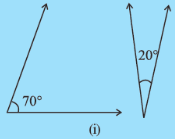Sum of the angles in the given figure is :

Thus two angles are complementary to each other.The sum of the two angles is :

In complementary angles sum of the angles is .  Hence given pair of angles are not complementary.We know that the sum angles of complementary angles is .

In the given figure:       Sum of angles is

Hence given pair of angles are not complementary.The sum of the two angles is :

In complementary angles sum of the angles is .  Hence given pair of angles are complementary to each other.

We know that the sum of complementary angles is .

Thus the complement of the given angle is :

The sum of complementary angles are .

Thus the required angle is :

We know that the sum of complementary angles is .

Hence the required complement of the given angle is :

We know that the sum of complementary angles is .

Hence the complement of the given angle is :

Let one of the angles be .

It is given that the angles are complementary to each other. So the other angle will be  .

Further, it is given that the difference of the angle is 12.

So the equation is :

or

or

Hence the two angles are   and  .

CBSE NCERT solutions for class 7 maths chapter 5 lines and angles topic 5.2.2

No, two obtuse angles cannot be supplementary as their the sum of angles will exceed .

No two acute angles cannot be supplementary.

For being the supplementary angles their sum should be .

But the acute angles are less than  . Hence their maximum doesn't reach .

Yes, two right angles are supplementary as their sum is .We know that the sum of the supplementary angle is .

(i)  Sum of the angles is :   .  Hence the angles are not supplementary.

(ii) Sum of the angles is :  .  Thus the angles are not supplementary.

(iii) Sum of the angles is :   . Hence the angles are supplementary to each other.

(iv) Sum of the angles is :   . Thus the angles are not supplement to each other.

Solutions of NCERT class 7 maths chapter 5 lines and angles topic 5.2.3

Yes, two adjacent angles can be supplementary.

For e.g.,   and    can be two adjacent angles which are supplementary angles.

Yes, two adjacent angles can be complementary to each other.

For e.g., adjacent angles  and  are complementary angles.

Yes, two obtuse angles can be adjacent for e.g.,   and  can be adjacent angles.

Yes, the acute angle can be adjacent to an obtuse angle.

For e.g.,  and   can be adjacent angles.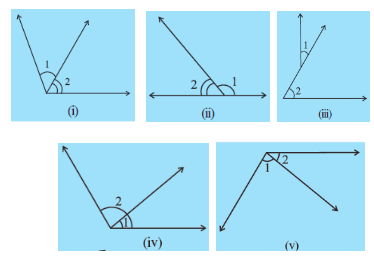The condition for being adjacent angles are:-

(a)  they have a common vertex

(b)  they have common arm

Hence in the given figures:-

(i)  These angles are adjacent angles as they agree above conditions.

(ii) The angles are adjacent angles.

(iii) These angles are not adjacent as their vertices are different.

(v) The angles are adjacent angles.

(a)   and
(b)   and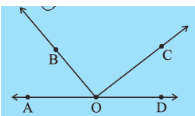(a)   and  are adjacent angles as they have common vertex and share a common arm.

(b)    and  are not adjacent angles as  is contained in .

Solutions for NCERT class 7 maths chapter 5 lines and angles topic 5.2.4

No two acute angles cannot form a linear pair. As the sum of angles in the linear pair is .

But the acute angles have their maximum value of  thus their sum cannot be .

No two obtuse angles cannot form a linear pair as their sum will exceed , but the sum of angles in linear pair is .

Yes, two right angles will form a linear pair as their sum is  which is the sum of angles in linear pair.The sum angles of linear pair is .

(i) Sum of the given angles is :   . Thus these are linear pair.

(ii) Sum of the given angles is :   . Thus these are not linear pair.

(iii) Sum of the given angles is :   . Thus these are not a linear pair.

(iv) Sum of the given angles is :   . Thus these are linear pair.

CBSE NCERT solutions for class 7 maths chapter 5 lines and angles topic 5.2.5From the given figure :

(a)       (Vertically opposite angles)

(b)         (Linear pair)

The very common example of vertically opposite angle is scissors. Its arms form vertically opposite angles.

Solutions of NCERT  class 7 maths chapter 5 lines and angles topic 5.3.1

The floor and the pillars in the house are at the right angle. Apart from this, the walls are perpendicular to the floor.

We know that the opposite sides of the rectangle are equal and parallel to each other.

Then for two interior angles on the same side of the transversal, we can write :

Also,                                          (Since opposite sides are equal)

Thus

No, it is not necessary that lines always intersect at right angles. The lines may form an acute angle (another angle will be obtuse as to form linear pair).

Solutions for class 7 maths chapter 5 lines and angles topic 5.3.2

We can draw infinite transversals from these two lines.

We know that transversal cuts lines at distinct points. Thus if a transversal cuts 3 lines then it will have 3 intersecting points.

Few examples of the transversal are road crossing of different railway line crossing the other lines. Transversal intersects lines at a distinct point.(ii)

The given pair of angles are alternate interior angles.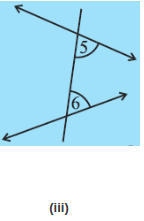The angles shown are pair of interior angles.(v)

The angles shown are pair of alternate interior angles.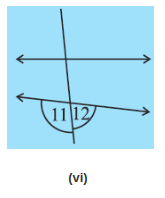The given angles are linear pair of angle as they form a straight line.

## NCERT solutions for class 7 maths chapter 5 lines and angles exercise 5.1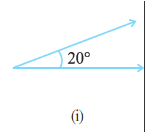The sum of the complementary angle is .

Thus the complementary angle to the given angle is :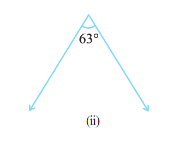The sum of the complementary angle is .

Thus the complementary angle to the given angle is :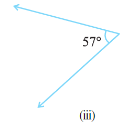The sum of the complement angles is .

Thus the complement of the angle is given by :

## 2.(i)   Find the supplement of each of the following angles: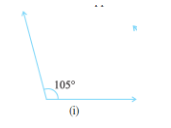We know that sum of supplement angles is

The supplement of the given angle is :We know that the sum of angles of supplementary pair is .

Thus the supplement of the given angle is :We know that the sum of angles of supplementary pair is .

Thus the supplement of the given angle is :

We know that the sum of supplementary angles is   and the sum of complementary angle is .

(i) Sum of the angles is :    . Hence these are supplementary angles.

(ii) Sum of the angles is :    . Hence these are complementary angles.

(iii) Sum of the angles is :    . Hence these are supplementary angles.

(iv) Sum of the angles is :    . Hence these are supplementary angles.

(v) Sum of the angles is :    . Hence these are complementary angles.

(vi)  Sum of the angles is :    . Hence these are complementary angles.

Let the required angle be .

Then according to question, we have :

or

or

Let the required angle be .

Then according to the question :

or

or

Hence the angle is .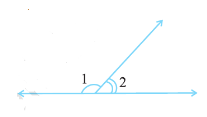Since it is given that  and  are supplementary angles, i.e. the sum of both angles is .

Thus if  is decreased then to maintain the sum  needs to be increased.

## 7.  Can two angles be supplementary if both of them are:

(i) acute ?   (ii) obtuse ?   (iii) right ?

We know that the sum of supplementary angles is .

(i) The maximum value of the sum of two acute angles is less than . Thus two acute angles can never be supplementary.

(ii) The minimum value of the sum of two obtuse angles is more than . Thus two obtuse angles can never be supplementary.

(iii) Sum of two right angles is . Hence two right angles are supplementary.

We know that the sum of two complementary angles is  .

Thus if one of the angles is greater than  then the other angle needs to be less than .

(iii) Do  and  form a linear pair?
(iv) Are  and  supplementary?
(v) Is  vertically opposite to  ?
(vi) What is the vertically opposite angle of  ?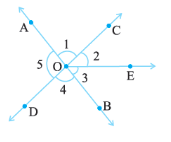(i) Yes,  adjacent to  as these have the same vertex and have one common arm.

(ii)  No,   is not adjacent to . This is because  contains .

(iii) Yes the given angles form a linear pair as they are pair of supplementary angles.

(iv) Since BOA is a straight line thus the given angles are supplementary.

(v) Yes,  and  are vertically opposite angles as they are the angles formed by two intersecting straight lines.

(vi) The vertically opposite angle to  is    .

(i) Vertically opposite angles.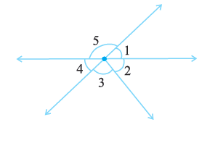The vertically opposite pairs are :

(a)    and

(b)    and

(ii) Linear pairs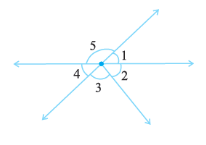The sum of angles in linear pair is .

Thus the linear pairs are :

(a)

(b)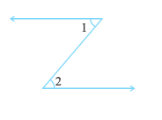No,   and    are not adjacent angles as their vertex is not same/common.

For being adjacent angles the pair must have a common vertex and have a common arm.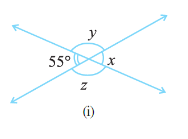From the figure :

(i)             (Vertically opposite angle)

(ii)      (Linear pair)

(iii)                     (Vertically opposite angle)From the figure we can observe that :

(i)                       (Vertically opposite angle)

(ii)             (Linear pair/straight line)

(iii)          (Vertically opposite angle).

(i) Obtuse vertically opposite angles
(iii) Equal supplementary angles
(iv) Unequal supplementary angles
(v) Adjacent angles that do not form a linear pair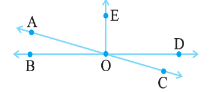(i)   are the vertically obtuse angles.

(ii)   are the complementary angles.

(iii)   are the equal supplementary angles.

(iv)   are the unequal pair of supplementary angle.

(v)  ,   and   are adjacent angles but are not supplementary angles.

## CBSE NCERT solutions for class 7 maths chapter 5 lines and angles exercise 5.2

(i) If  , then .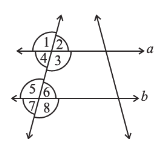The statement  "If  , then   "  is true using the corresponding angles property.

(ii) If  , thenThe property used here is 'alternate interior angle property'.

(iii) If  , thenThe property used here is 'Interior angles on the same side of the transversal are a pair of supplementary angles'.

## 2.  In the adjoining figure, identify

(i) the pairs of corresponding angles.
(ii) the pairs of alternate interior angles.
(iii) the pairs of interior angles on the same side of the transversal.
(iv) the vertically opposite angles.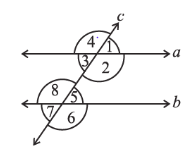(i) Corresponding angles :-    ,  ,  ,

(ii) Alternate interior angles :-    ,  ,

(iii) Alternate angles on the same side of traversal :-   ,

(iv) Vertically opposite angles :-       ,  ,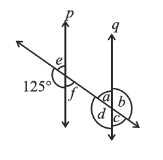The angles can be found using different properties:

(a)         (The angles are linear pair)

(b)                              (Vertically opposite angle)

(c)                                            (Corresponding angle)

(d)                             (Vertically opposite angle)

(e)              (Vertically opposite angel,  linear pair).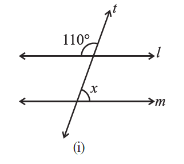The linear pair of the  is :

Thus the value of x is :                                        (Corresponding angles of parallel lines are equal).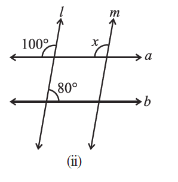The value of x is ,  as these are the corresponding angles.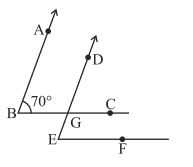(i) Since side AB is parallel to DG.

Thus :                           (Corresponding angles of parallel arms are equal.)

(ii)     Further side BC is parallel to EF.

We have :                             (Corresponding angles of parallel arms are equal.)(i) In this case. the sum of the interior angle is  thus l is not parallel to m.\

(ii) In this case also l is not parallel to m as the corresponding angle cannot be   (Linear pair will not form).

(iii) In this l and m are parallel. This is because the corresponding angle is  and it forms linear pair with   .

(iv) The lines are not parallel as the linear pair not form. (Since the corresponding angle will be  otherwise.)

## NCERT Solutions for Class 7 Maths - Chapter-wise

 Chapter No. Chapter Name Chapter 1 Solutions of NCERT for class 7 maths chapter 1 Integers Chapter 2 CBSE NCERT solutions for class 7 maths chapter 2 Fractions and Decimals Chapter 3 NCERT solutions for class 7 maths chapter 3 Data Handling Chapter 4 Solutions of NCERT for class 7 maths chapter 4 Simple Equations Chapter 5 CBSE NCERT solutions for class 7 maths chapter 5 Lines and Angles Chapter 6 NCERT solutions for class 7 maths chapter 6 The Triangle and its Properties Chapter 7 Solutions of NCERT for class 7 maths chapter 7 Congruence of Triangles Chapter 8 NCERT solutions for class 7 maths chapter 8 comparing quantities Chapter 9 CBSE NCERT solutions for class 7 maths chapter 9 Rational Numbers Chapter 10 NCERT solutions for class 7 maths chapter 10 Practical Geometry Chapter 11 Solutions of NCERT for class 7 maths chapter 11 Perimeter and Area Chapter 12 CBSE NCERT solutions for class 7 maths chapter 12 Algebraic Expressions Chapter 13 NCERT solutions for class 7 maths chapter 13 Exponents and Powers Chapter 14 Solutions of NCERT for class 7 maths chapter 14 Symmetry

## Benefits of  NCERT solutions for class 7 maths chapter 5 lines and angles:

• Solving homework is an easy task with solutions of NCERT class 7 maths chapter 5 lines and angles in hand.
• Questions of similar type from CBSE NCERT solutions for class 7 maths chapter 5 are expected for the class exams.
• Practice all the questions from NCERT solutions for class 7 maths chapter 5 lines and angles to score well in the exam.